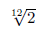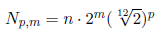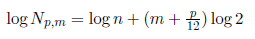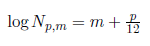Musicians are rarely interested in mathematics; most of them do not feel respect for this science, try to keep as far as possible from it. Meanwhile, musicians - even those who do not believe, like Salieri in Pushkin in "harmonic algebra" are confronted with mathematics much more often than they suspect, also with such "scary things" like logarithms. Let me quote a passage from the article on the subject of the Russian physicist Prof. A. Eichenwald:

"My junior high school friend liked to play the piano, but he did not like mathematics. He even spoke with a shade of contempt that music and mathematics has nothing to do with each other. Admittedly, Pythagoras found some relations between voice vibrations, but the Pythagorean range did not find any application in our music world. Just imagine how unpleasantly surprised my friend was when I proved to him that playing on the keys of a contemporary piano, he plays, in fact, based on ... logarithms."

Indeed, the so-called "grades" of the tempered chromatic range are not spaced at equal distances either in terms of the number of vibrations, or in terms of the wavelength of the corresponding tones, but they are represent by the logarithms of these quantities. Only the base of these logarithms is equal to 2, not 10, as accepted in other cases.

Let us assume that the note "do" the lowest octave - we call it the octave zero - corresponds to n vibrations per second. Then "do" of the first octave corresponds to 2n vibrations, and "do" of m-octave n * 2m vibrations, etc. We will mark all the notes of the chromatic piano range with the p-numbers, assuming the fundamental tone to each octave as zero; then, for example, the tone of "sol" will be seventh, and "la" will be ninth, etc .; 12th ton will be "do" again, only an octave higher. Due to the fact that in the tempered chromatic range each next tone hasgreater number of vibrations than the previous ones, the number of vibrations of any tone can be expressed by the formula:The logarithm of the formula, is:orAnd assuming the number of vibrations to "do" for one (n = 1) and bringing all logarithms to the basis of 2 (or just accepting log 2 = 1), we have:Thus, we can see that the key numbers represent the logarithms of the vibrations of the corresponding sounds (multiply by 12). We can even say that the octave numbers represent the characteristic of a logarithm, the number of the sound in a given octave (divided by 12) - the mantissa of this logarithm.
For example:

In the "sol" tone of the third octave,  3 + 7/12 (= 3.583), the number 3 is the logarithm of the number of vibrations of this tone, and 7/12 (= 3,583) is the mantissa of this logarithm with base 2; the number of vibrations is therefore               2^(3.583) - it is 11.98 times greater than the number of vibrations "do" of the first octave.